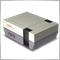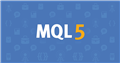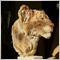# rectangle draw by hand return X Y + position426

hi guys  if i draw  by hand  a rectangle  is  possible by script   have  a coordinate in chart  and dimention ??

anyone  have some example  thankz24207

1. "Draw by hand" is irrevalent.
2. There are no such things with a rectangle.
3. Rectangle coordinates are price and time. ObjectGetDouble/OBJPROP_PRICE

Perhaps you should read the manual.
How To Ask Questions The Smart Way. 2004
RTFM and STFW: How To Tell You've Seriously Screwed Up.426

Thankz  i saw

i create somthing like this  but i dont can find  a time coordinate

```int start()
{
for(int i = ObjectsTotal(); i >= 0; i--)
{

string name = ObjectName(i);

if(ObjectType(name) == OBJ_RECTANGLE )
{
double price1 =        ObjectGetDouble(MainWINDOW, name, OBJPROP_PRICE1);
double price2 =        ObjectGetDouble(MainWINDOW, name, OBJPROP_PRICE2);
datetime time1 =        ObjectGetTimeByValue(MainWINDOW, name,price1, 0);
datetime time2 =        ObjectGetTimeByValue(MainWINDOW, name, OBJPROP_TIME2);
color  rColor = color(ObjectGetInteger(MainWINDOW, name, OBJPROP_COLOR));
Print("babbabbba "+ TimeToStr(time1));
Print("babbabbba222 "+ TimeToStr(time2));

}
}

return 0;
}
```

where is  the problem ???Documentation on MQL5: Constants, Enumerations and Structures / Objects Constants / Object Properties
• www.mql5.com
All objects used in technical analysis are bound to the time and price coordinates: trendline, channels, Fibonacci tools, etc. But there is a number of auxiliary objects intended to improve the user interface that are bound to the always visible part of a chart (main chart windows or indicator subwindows): – defines the chart corner relative to...24207

faustf: where is  the problem ???
`if(ObjectType(name) == OBJ_RECTANGLE )`

Perhaps you should read the manual.
How To Ask Questions The Smart Way. 2004
RTFM and STFW: How To Tell You've Seriously Screwed Up.

An object can have several values in one price coordinate, therefore it is necessary to specify the line number. This function applies only to the following objects: Trendline (OBJ_TREND) Trendline by angle (OBJ_TRENDBYANGLE) Gann line (OBJ_GANNLINE) Equidistant channel (OBJ_CHANNEL) - 2 lines Linear regression channel (OBJ_REGRESSION) - 3 lines Standard deviation channel (OBJ_STDDEVCHANNEL) - 3 lines426

thankz  i resolve  with this   code

```         double price1 =        ObjectGetDouble(0, name, OBJPROP_PRICE1,0);
double price2 =        ObjectGetDouble(0, name, OBJPROP_PRICE2,0);
datetime time1 =        ObjectGet(name,OBJPROP_TIME1);
datetime time2 =        ObjectGet(name,OBJPROP_TIME2);```547

` for(int i = ObjectsTotal(); i >= 0; i--)`

You also have a mistake in the loop, it should be one of the below cases:

``` for(int i = ObjectsTotal()-1; i >= 0; i--)

for(int i = ObjectsTotal(); i > 0; i--)```24207

` for(int i = ObjectsTotal(); i > 0; i--)`

That will not work, you will still iterate with one invalid index and miss index zero.

1. ``` for(int i = ObjectsTotal(); i > 0; i--) {
string name = ObjectName(i-1);
```
works, as does
2. ``` for(int i = ObjectsTotal(); i > 1; ) { i--;
string name = ObjectName(i);```Moderator
27970

Reza nasimi:

You also have a mistake in the loop, it should be one of the below cases:

```for(int i = ObjectsTotal()-1; i >= 0; i--)

for(int i = ObjectsTotal(); i > 0; i--)```
The second one is wrong.547

Oh, sorry, what a mistake.